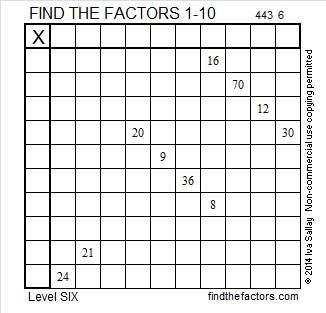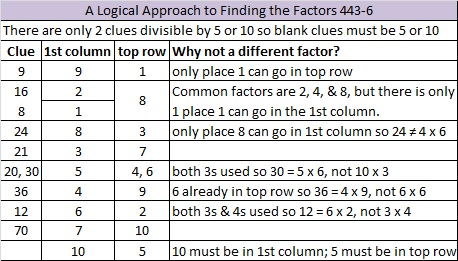# 283 and Level 6

• 283 is a prime number.
• Prime factorization: 283 is prime.
• The exponent of prime number 283 is 1. Adding 1 to that exponent we get (1 + 1) = 2. Therefore 283 has exactly 2 factors.
• Factors of 283: 1, 283
• Factor pairs: 283 = 1 x 283
• 283 has no square factors that allow its square root to be simplified. √283 ≈ 16.823How do we know that 283 is a prime number? If 283 were not a prime number, then it would be divisible by at least one prime number less than or equal to √283 ≈ 16.823. Since 283 cannot be divided evenly by 2, 3, 5, 7, 11, or 13, we know that 283 is a prime number.Print the puzzles or type the factors on this excel file: 10 Factors 2014-10-27This site uses Akismet to reduce spam. Learn how your comment data is processed.